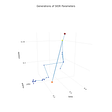# How to create a bar chart from two columns in a Pandas DataFrame?

`import pandas as pdimport matplotlib.pyplot as pltdf = pd.DataFrame({"X": ['Jan', 'Feb', 'Mar', 'Apr', 'May', 'Jun'], "Y": [50, 73, 68, 47, 60, 65]})`

The DataFrame looks as follows:

`df.plot(x = "X", y = "Y", kind = "bar")plt.show()`

The plot looks as follows:

You can remove the legend from the above as follows.

`#exclude legenddf.plot(x = "X", y = "Y", kind = "bar", legend = False)plt.show()`

Here’s the code in github:

--

--

--

## More from Digestize

#probability #statistics #ML #DL #coding #security #building #digest

Love podcasts or audiobooks? Learn on the go with our new app.

## Deep Diving for Data Analytics## How Prediction Markets Work## How To Build a Recommender System Using Netflix Data## Using genetic algorithm for COVID-19 data analysis## How to remove unbrushed data of plot in MatLab?## Digestize

#probability #statistics #ML #DL #coding #security #building #digest

## [TUTORIAL] A BASIC DATA ANALYSIS WITH POSITIVES COVID 19 PERU USING PYTHON## Pandas dtypes: To category or not to category?## Introduction to Python(Part-2): Python Installation